Courses
Courses for Kids
Free study material
Free LIVE classes
More

# NCERT Solutions for Class 6 Maths Chapter 9 - Data HandlingLIVE
Join Vedantu’s FREE Mastercalss

## NCERT Solutions for Class 6 Maths Chapter 9 - Data Handling

Students who are looking for a reference book to aid them in their exam preparations, they can refer to NCERT Solutions for Class 6 Maths Chapter 9. It offers accurate and comprehensive answers to the exercise questions and helps candidates to improve their preparations, and subsequently, their final marks. Download the free Data Handling in Maths Class 6 NCERT Solutions PDF and start preparing from today. You can also register Online for NCERT Solutions Class 6 Science tuition on Vedantu.com to score more marks in your examination.

### List of Topics Covered Under NCERT Solutions for Class 6 Math Chapter 9

 Introduction Recording Data Organization of Data Pictograph Interpretation of Pictograph Drawing a Pictograph Bar Graph

### A Glance About The Topic

• It is impossible to process the vast amount of data without a proper standard.  The process of raw information is known as Data.

• The organized data can be represented as a picture in the form of a bar graph or pie chart.

• To compare two or more same sets of data can be drawn pictorially by using a bar graph.

• The pictographs can also represent using some symbols

• In the bar graph, the value of 1 unit can be scaled according to the need.

Last updated date: 15th Sep 2023
Total views: 453k
Views today: 6.53k

## Access NCERT Solutions for Class 6 Chapter 9 – Data Handling

Exercise – 9.1

1. In a mathematics test the following marks were obtained by $40$ students. Arrange these marks in a table using tally marks.

 8 1 3 7 6 5 5 4 4 2 4 9 5 3 7 1 6 5 2 7 7 3 8 4 2 8 9 5 8 6 7 5 5 6 9 6 4 4 6 6

(a) Find how many students obtained marks equal to or more than $7$

(b) How many students obtained marks below $4$?

Ans:

 Marks Tally Marks No. Of Students $1$ $||$ 2 2 ||| 3 3 ||| 3 4 $\require{cancel}\cancel{||||}$| 6 5 $\require{cancel}\cancel{||||}$|| 7 6 $\require{cancel}\cancel{||||}$|| 7 7 $\require{cancel}\cancel{||||}$ 5 8 |||| 4 9 ||| 3

(a) There are twelve students who obtained marks equal to more than $7$ .

(b) There are eight students who scored less than $4$.

2. Following is the choice of sweets for $30$ students of Class VI.

(a) Arrange the names of sweets in a table using tally marks.

(b) Which sweet is preferred by most of the students?

Ans:

(a)

 Sweets Tally Marks No. of students Ladoo $\require{cancel}\cancel{||||}$$\require{cancel}\cancel{||||} | 11 Barfi ||| 3 Jalebi \require{cancel}\cancel{||||}|| 7 Rasgulla \require{cancel}\cancel{||||} |||| 9 Total 30 (b) The sweet which is most preferred is Ladoo. 3. Catherine threw a dice 40 times and noted the number appearing each time as shown below:  1 3 5 6 6 3 5 4 1 6 2 5 3 4 6 1 5 5 6 1 1 2 2 3 5 2 4 5 5 6 5 1 6 2 3 5 2 4 1 5 --- --- Make a table and enter the data using tally marks. Find the number that appeared. (a) The minimum number of times (b) The maximum number of times. (c) Find those numbers that appear an equal number of times. Ans:  Numbers Tally Marks How many times? 1 \require{cancel}\cancel{||||}|| 7 2 \require{cancel}\cancel{||||}| 6 3 \require{cancel}\cancel{||||} 5 4 |||| 4 5 \require{cancel}\cancel{||||}$$\require{cancel}\cancel{||||}$| 11 6 $\require{cancel}\cancel{||||}$|| 7

(a) The minimum number of times is $4$

(b) The maximum number of times is $5$ .

(c) The number that appear an equal number of times are $1,6$

4. Following pictograph shows the number of tractors in five villages: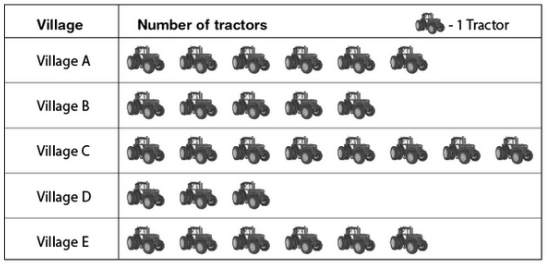Observe the pictograph and answer the following questions:

(i) Which village has the minimum number of tractors?

(ii) Which village has the maximum number of tractors?

(iii) How many more tractors village C has as compared to village B.

(iv) What is the total number of tractors in all the five villages?

Ans:

(i) Village D has a minimum number of tractors.

(ii) Village C has a maximum number of tractors.

(iii) Village C has $3$ more tractors as compared to village B.

(iv) Total number of tractors in all five villages is $28$ .

5. The number of girl students in each class of a co-educational middle school is depicted by the pictograph.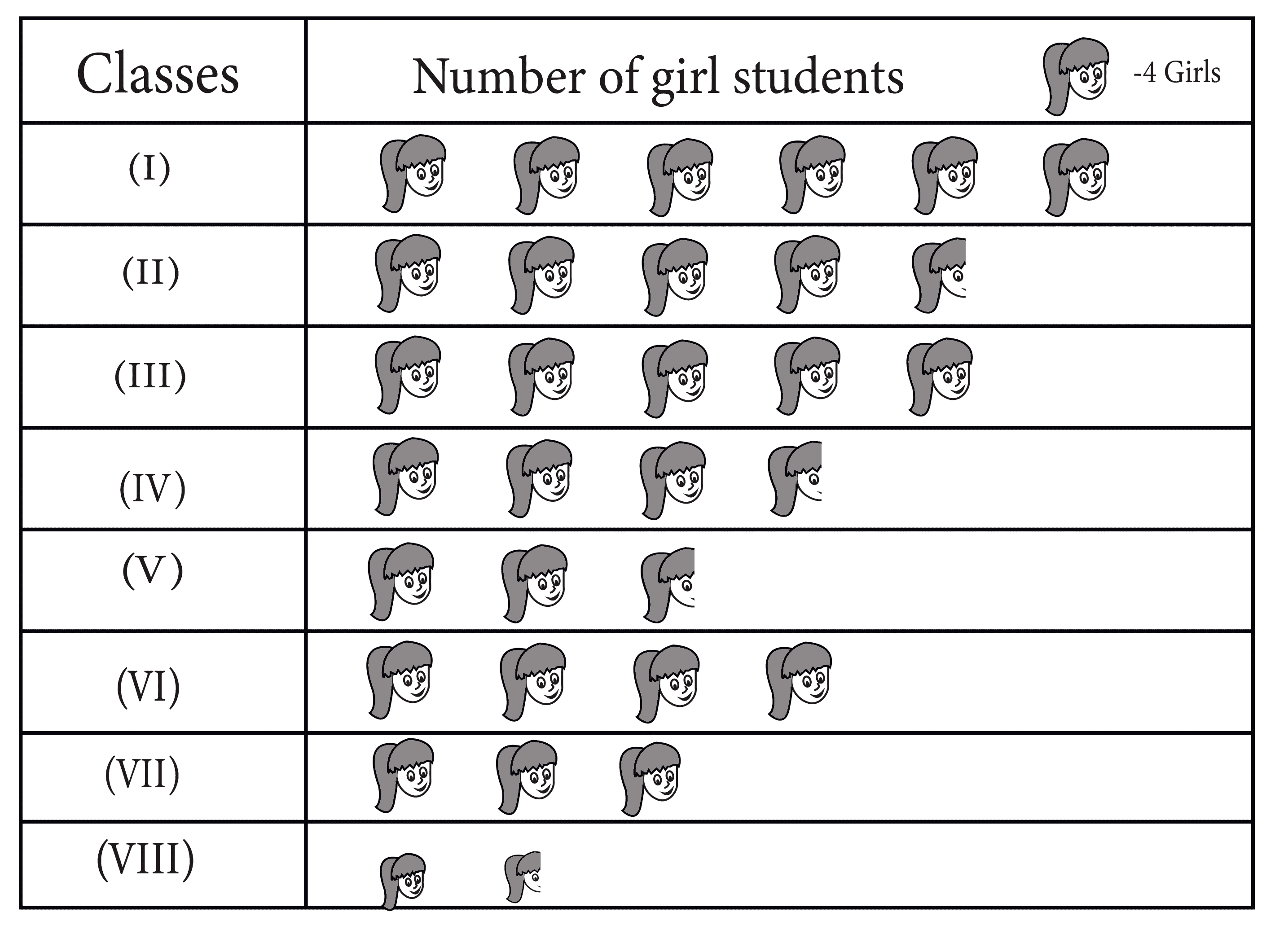Observe this pictograph and answer the following questions:

(a) Which class has the minimum number of girl students?

(b) Is the number of girls in class VI less than the number of girls in class V?

(c) How many girls are there in class VII?

Ans:

(a) Class VIII has a minimum number of girl students.

(b) No, the number of girls in class VI is not less than the number of girls in class V

(c) There are twelve girls in class eight i.e. $3 \times 4 = 12$ girls

6. The sale of electric bulbs on different days of a week is shown below: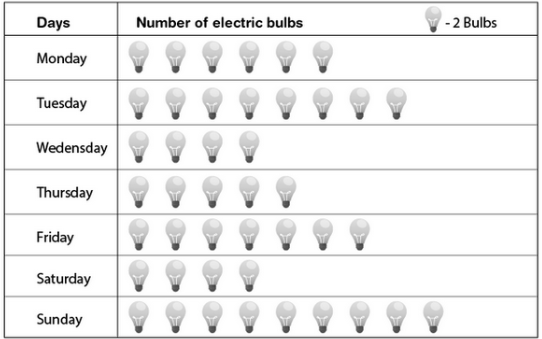What can be concluded from the above pictograph?

Ans: From the above pictograph it can be concluded that the maximum number of bulbs were sold on Sunday and the total numbers of bulbs sold in the given week were $86$ .

7. In a village six fruit merchants sold the following number of fruit baskets in a particular season: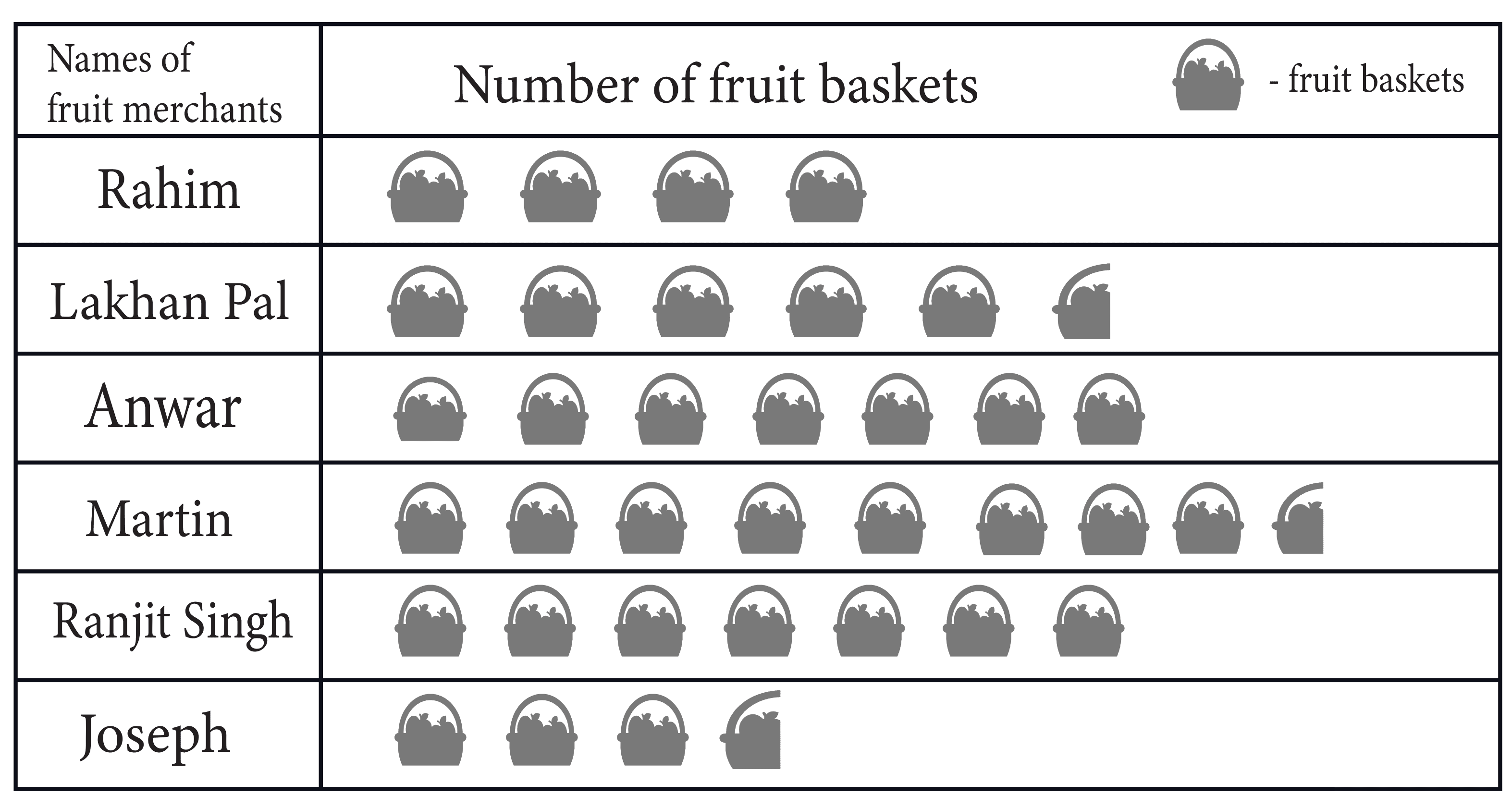Observe this pictograph and answer the following questions:

(a) Which merchant sold the maximum number of baskets?

(b) How many fruit baskets were sold by Anwar?

(c) The merchants who have sold $600$ or more number of baskets are planning to buy a godown for the next season. Can you name them?

Ans:

(a) Martin sold the maximum number of baskets.

(b) Total number of baskets sold by Anwar are $7 \times 100 = 700$ fruit baskets.

(c) Name of them are- Anwar, Martin and Ranjit Singh

Exercise – 9.2

Refer to page 6 - 8 for exercise 9.2 in the PDF

1. Total number of animals in five villages are as follows:

 Village A $80$ Village B $120$ Village C $90$ Village D $40$ Village E $60$

Prepare a pictograph of these animals using one symbolto represent $10$ animals and answer the following questions:

(a) How many symbols represent animals of village E?

(b) Which village has the maximum number of animals?

(c) Which village has more animals: village A or village C?

Ans: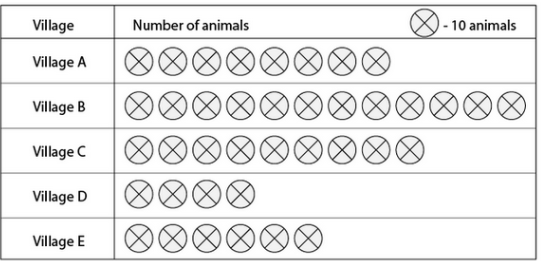(a) There are $6$ symbols that represent animals of Village E.

(b) Village B has a maximum number of animals.

(c) From the above pictograph, village C has more animals than village A.

2. Total number of students of a school in different years is shown in the following table:

 Years Number of students 1996 400 1998 535 2000 472 2002 600 2004 623

A. Prepare a pictograph of students using one symbol  (Image will be uploaded soon) to represent 100 students and answer the following questions:

(a) How many symbols represent total number of students in the year $2002?$

(b) How many symbols represent the total number of students for the year $1998$?

B. Prepare another pictograph of students using any other symbol each representing $50$ students. Which pictograph do you find more informative?

Ans:

A.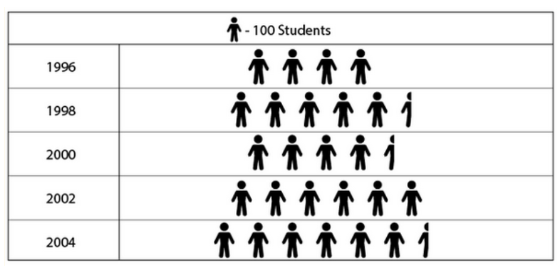(a) There are $6$ symbols that represent the total number of students in the year $2002$ .

(b) There are $5$ and half of scale  symbols that represent total number of students for the year $1998$

B.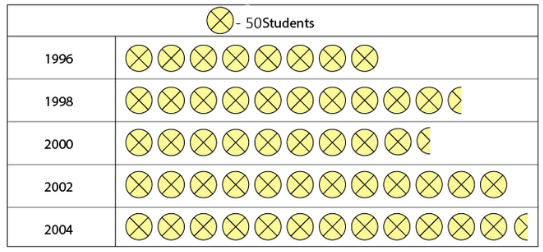Exercise – 9.3

1. The bar graph given below shows the amount of wheat purchased by government during the year $1998 - 2002$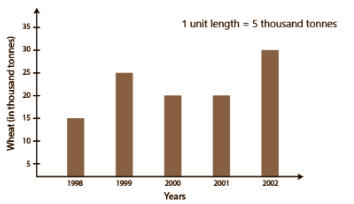(a) In which year was the wheat production maximum?

(b) In which year was the wheat production minimum?

Ans:

(a) In the year $2002$ , there was maximum wheat production.

(b) In the year $1998$ , there was the minimum wheat production.

2. Observe this bar graph which is showing the sale of shirts in a readymade shop from Monday to Saturday.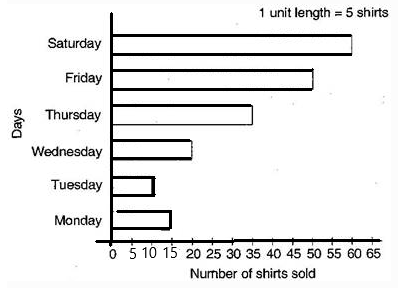(a) What information does the above bar graph give?

(b) What is the scale chosen on the horizontal line representing the number of shirts?

(c) On which day were the maximum number of shirts sold? How many shirts were sold on that day?.

(d) On which day were the minimum number of shirts sold?

(e) How many shirts were sold on Thursday?

Ans:

(a) The above bar graph gives the information of the sale of shirts in a readymade shop from Monday to Saturday.

(b) The chosen scale on the horizontal line representing number of shirts is

$1$ unit $= 5$ shirts.

(c) The maximum  number of shirts are sold on Saturday. There were a total of $60$ shirts sold on that day.

(d) On Tuesday there were a minimum number of shirts sold.

(e) On Thursday, $35$ shirts were sold.

3. Observe this bar graph which shows the marks obtained by Aziz in half yearly examination in different subjects: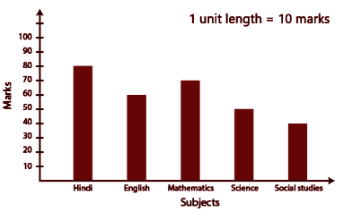(a) What information does the bar graph give?

(b) Name the subject in which Aziz scored maximum marks.

(c) Name the subject in which he has scored minimum marks.

(d) State the name of the subjects and marks obtained in each of them.

Ans:

(a) The above bar graph gives information of the  marks obtained by Aziz in half yearly examinations in different subjects.

(b) Aziz scored maximum marks in Hindi.

(c) Aziz scored minimum marks in Social Studies.

(d) The name of the subjects are:  Hindi $- 80$, English $- 60$, Mathematics$- 70$, Science $- 50$ , Social Studies$- 40$

Exercise – 9.4

1. A survey of 120 school students was done to find which activity they prefer to do in their free time:

 Preferred activities Number of students Playing 45 Reading story books 30 Watching TV 20 Listening to music 10 Painting 15

Draw a bar graph to illustrate the above data taking a scale of $1$ unit length $= 5$ students. Which activity is preferred by most of the students other than playing?

Ans:

By taking a scale of $1$ unit length $= 5$ students, bar graph is shown below considering the above data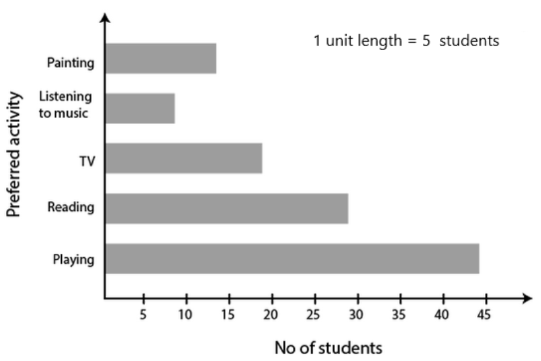2. The number of mathematics books sold by a shopkeeper on six consecutive days is shown below:

 Days Sunday Monday Tuesday Wednesday Thursday Friday Number of books sold 65 40 30 50 20 70

Draw a bar graph to represent the above information choosing the scale of your choice.

Ans:

The bar graph of the above given data is as follows: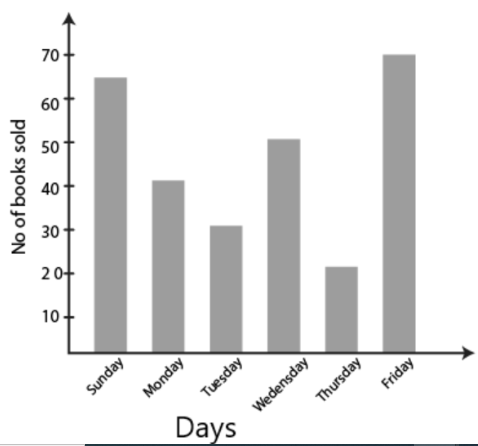3. Following shows the number of bicycles manufactured in a factory during the year 1998 to 2002. Illustrate this data using a bar graph. Choose a scale of your choice.

 Years Number of bicycles manufactured 1998 800 1999 600 2000 900 2001 1100 2002 1200

(a) In which year were the maximum number of bicycles manufacturers?

(b) In which year were the minimum number of bicycles manufactured?

Ans:

By taking a scale of $1$ unit length $= 100$ bicycles we may draw a bar graph of above data as follows: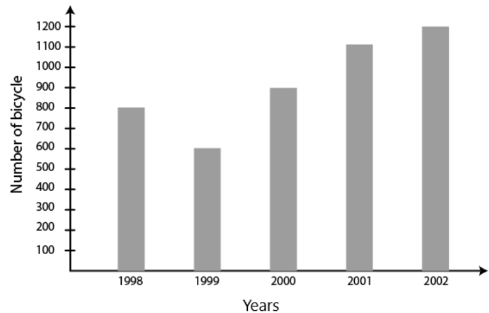(a) The maximum number of bicycles are manufactured in the year $2002$

(b) The minimum number of bicycles are manufactured in the year $1999$

4. Number of persons in various age groups in a town is given in the following table:

 Age group Number of persons 1-14 2 lakhs 15-29 1 lakh 60 thousand 30-44 1 lakh 20 thousand 45-59 1 lakh 20 thousand 60-74 80 thousands 75 and above 40 thousands

Draw a bar graph to represent the above information and answer the following questions. (take $1$ unit length $= 20$ thousands)

(a) Which two age groups have the same population?

(b) All persons in the age group of $60$ and above are called senior citizens.

How many senior citizens are there in the town?

Ans:

From the question, by taking a scale of $1$ unit length $= 20$ thousands.

The bar graph of above data as follows: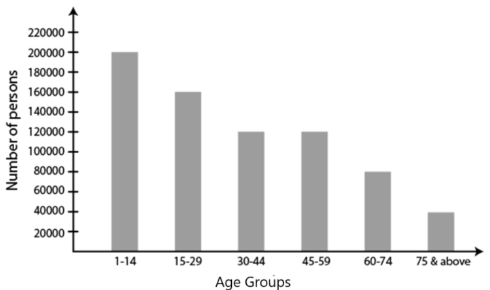(a) The two age groups which have same population are $30 - 44$ and $45 - 59$

(b) Senior citizens are the persons between the age group either from $60 - 74$ or from $75$above. Therefore number of senior citizens in the town

$80000 + 40000 = 1$ lakh $20$ thousand

Hence, the number of senior citizens in the town is $1$ lakh $20$ thousand.

Chapter number nine of NCERT class 6 mathematics presents the concept of data handling. In this chapter students learn the concept of recording data, organising, and managing them.

Data handling is a complex concept, and Class 6 Maths Chapter 9 aims to simplify it. Understanding this concept requires conceptual knowledge, and this chapter intends to provide the same. It begins with an introduction to data and its application in daily life. There are a few real-life examples as well. After that, it gradually introduces the vital concepts like data recording, organising, etc. Along with these, candidates also get to learn more about pictograph, its interpretation, and the process of drawing one. They further come across various bar graphs as well in this chapter.

NCERT Solutions for Class 6 Maths Chapter 9 is available for free online in PDF format. Students can easily download them to enhance their exam preparations.

### NCERT Solutions For Class 6 Maths Chapter 9 Data Handling – Topic - Wise Discussion

NCERT maths Class 6 Chapter 9 has a total of seven sub-topics. Here is a topic-wise brief:

1. Introduction

The introduction on Data Handling for Class 6 chapter begins with an introduction to data and its real-world applications.

2. Recording Data

Recording data is an essential part of daily life. Data handling Class 6 further explains this process. There are various processes of recording data and in this particular section students come across a specific process.

Here, an example has been used to simplify this process. A class picnic is taking place, and the teacher asks everyone’s name and their preferred fruit. The entire data is collected in two different methods, but one of them seems more manageable than the other.

This example presented in Data Handling Class 6 PDF; students learn how collecting data randomly can haphazard the entire process. It also prompts students to perform this example in real-life to understand it better.

3. Organisation of Data

Recording of data can be tiresome, if not organised properly. In this regard, students should know about this concept. Data handling for Class 6 explains this concept further. This section takes a queue from the previous example for this purpose and shows how to organise data.

Along with that, another example shows that a teacher wants to collect data regarding food preference for the mid-day meal programme. She assigns this task to one of her students. She uses columns to represent the choices, but another student suggested a better method of classifying that data. There are two more ways mentioned here that candidates can learn to segregate any data.

This section in NCERT Maths book Class 6 Chapter 9 solutions contains two more examples regarding the same topic. Students can study them thoroughly to learn more about data organisation.

4. Pictograph

Pictograph helps to identify data via visual representation. NCERT solution for Class 6 Maths chapter 9 introduces the topic of pictograph with a representation of a bookshelf. Students can quickly identify the number of books from this visual representation.

5. Interpretation of Pictograph

In this section of NCERT Class 6 Maths Data Handling students learn how to interpret data efficiently.

There are multiple examples in this section with a visual representation of data. It is then followed by questions which students need to answer by taking reference from the pictogram above. They can review their answers from the solutions provided immediately below the questions, to evaluate if they have understood the concept or not. In case of any difficulties, candidates must take the assistance of their teachers to resolve their queries.

NCERT solutions Class 6 Maths Chapter 9 offers a comprehensive solution to the following exercise.

6. Drawing a Pictograph

Class 6 Maths Ch 9 moves on to the topic of drawing a pictograph. Even though it is not hard to create a pictograph, some pictures can be hard to draw, if it represents multiple units. Thereby, students can opt for different symbols for this purpose. There are various examples and exercises in this section as well that help students further in study.

7. A Bar Graph

Even though pictograph is a valid option, representing data via them can be a time-consuming affair. Bar graphs can be a good alternative here. In this section of Class 6th Maths Chapter 9 candidates get an introduction to this method, and how it can save more time. There are two additional sub-sections under this topic, which are:

• Interpretation of bar graph.

• Drawing a bar graph.

### Improve the Exam Preparations with NCERT Solutions

NCERT solutions for Class 6 Maths Chapter 9 help students to improve their exam preparations with its following features:

• NCERT solutions use simple language that helps students to comprehend the topics easily.

• They abide by the curriculum of CBSE helping students to keep a track of their preparations.

• Since subject experts are in charge of these study materials, they are accurate and error-free.

• These NCERT solutions offer an in-depth analysis of different topics, eliminating the need for any additional reference book.

NCERT solutions for Class 6 Maths Chapter 9 are available online for free. Students can download them in PDF format from the official website of Vedantu – India’s leading e-learning platform.

### Chapter wise NCERT Solutions for Class 6 Maths

Along with this, students can also view additional study materials provided by Vedantu, for Class 6 Maths Chapter 1 Knowing Our Numbers

### NCERT Solution Class 6 Maths of Chapter 9 All Exercises

Chapter 9 - Data Handling Exercises in PDF Format

Exercise 9.1

7 Questions with Solutions

Exercise 9.2

1 Questions with Solutions

Exercise 9.3

2 Questions with Solutions

## FAQs on NCERT Solutions for Class 6 Maths Chapter 9 - Data Handling

1. How Many Topics are There in the NCERT Solution Class 6 Maths Chapters 9 Introduction on Data Handling for Class Solutions for Class 6 Maths Chapter 9 PDF?

There are a total of seven topics in this chapter, and each of them describes a vital concept of data. It begins with a general introduction to the topic and moves on to the concepts of recording and organising data. It further describes the idea of pictograph and its usage and how one can benefit from using it. After that, students get to learn about bar graphs and how they can help to identify data faster and accurately. They understand the techniques of drawing a bar graph efficiently from this chapter as well.

2. What is Data handling for Class 6th?

Data handling is the collection of some kind of data and arranging it in a proper format to understand it easily. Students of Class 6 study Data Handling in Chapter 9. They can find NCERT Solutions on Vedantu. NCERT Solutions can help students to learn and understand the data handling concepts and solve the NCERT textbook questions easily. The solutions provided by Vedantu are free of cost. They are also available on the Vedantu Mobile app.

3. What is included in Data Handling Class 6 Maths Chapter 9?

Data handling includes various concepts. Students will learn how to arrange the given data in order and find the mean, median, frequency, and mode of the given data. There are different types of data collection and arrangement. It helps students to arrange the raw data in an organized manner. They will also learn about bar graphs, pie charts, and other forms of data handling.

4. How many types of data handling are there?

Data may be available in two main forms: Qualitative data and quantitative data. Data handling includes learning about different concepts of data. They will learn about bar graphs, line graphs, stem and leaf plots, histograms, pie charts, etc. In Class 6 Maths, students can understand the different concepts of data handling from the notes given at Vedantu. All the notes are given by the expert subject teachers.

5. How do you arrange the given raw data?

Raw data can be arranged in different ways. If the data is given in the form of numerical numbers, students can arrange the numbers in ascending order for easy understanding. The given data can be represented in the form of bar graphs, line graphs, pie charts, or stem and leaf plots. Students will learn about data handling in Class 6 Maths Chapter 9. They can download NCERT Solutions for Class 6  Chapter 9 for an easy and quick understanding of the concepts of data handling.